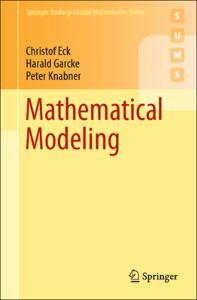Please use this identifier to cite or link to this item: `http://localhost/handle/Hannan/413`
 Title: Mathematical Modeling Authors: Eck, Christof. ;;Garcke, Harald. ;;Knabner, Peter. ; subject: Mathematics. ;;Mathematical models. ;;Mathematics. ;;Mathematical Modeling and Industrial Mathematics. ; Year: 2017 place: Cham : Publisher: Springer International Publishing :Imprint: Springer, Series/Report no.: Springer Undergraduate Mathematics Series, ; 1615-2085. ;Springer Undergraduate Mathematics Series, ; 1615-2085. ; Abstract: Mathematical models are the decisive tool to explain and predict phenomena in the natural and engineering sciences. With this book readers will learn to derive mathematical models which help to understand real world phenomena. At the same time a wealth of important examples for the abstract concepts treated in the curriculum of mathematics degrees are given. An essential feature of this book is that mathematical structures are used as an ordering principle and not the fields of application. Methods from linear algebra, analysis and the theory of ordinary and partial differential equations are thoroughly introduced and applied in the modeling process. Examples of applications in the fields electrical networks, chemical reaction dynamics, population dynamics, fluid dynamics, elasticity theory and crystal growth are treated comprehensively. ; Description: Printed edition: ; 9783319551609. ;SpringerLink (Online service) ; URI: http://localhost/handle/Hannan/413 ISBN: 9783319551616 ;9783319551609 (print) ; More Information: XV, 509 p. 107 illus., 2 illus. in color. ; online resource. ; Appears in Collections: مهندسی مدیریت ساخت

Files in This Item:
File Description SizeFormat
9783319551616.pdf6.92 MBAdobe PDFTitle: Mathematical Modeling Authors: Eck, Christof. ;;Garcke, Harald. ;;Knabner, Peter. ; subject: Mathematics. ;;Mathematical models. ;;Mathematics. ;;Mathematical Modeling and Industrial Mathematics. ; Year: 2017 place: Cham : Publisher: Springer International Publishing :Imprint: Springer, Series/Report no.: Springer Undergraduate Mathematics Series, ; 1615-2085. ;Springer Undergraduate Mathematics Series, ; 1615-2085. ; Abstract: Mathematical models are the decisive tool to explain and predict phenomena in the natural and engineering sciences. With this book readers will learn to derive mathematical models which help to understand real world phenomena. At the same time a wealth of important examples for the abstract concepts treated in the curriculum of mathematics degrees are given. An essential feature of this book is that mathematical structures are used as an ordering principle and not the fields of application. Methods from linear algebra, analysis and the theory of ordinary and partial differential equations are thoroughly introduced and applied in the modeling process. Examples of applications in the fields electrical networks, chemical reaction dynamics, population dynamics, fluid dynamics, elasticity theory and crystal growth are treated comprehensively. ; Description: Printed edition: ; 9783319551609. ;SpringerLink (Online service) ; URI: http://localhost/handle/Hannan/413 ISBN: 9783319551616 ;9783319551609 (print) ; More Information: XV, 509 p. 107 illus., 2 illus. in color. ; online resource. ; Appears in Collections: مهندسی مدیریت ساخت

Files in This Item:
File Description SizeFormat
9783319551616.pdf6.92 MBAdobe PDFTitle: Mathematical Modeling Authors: Eck, Christof. ;;Garcke, Harald. ;;Knabner, Peter. ; subject: Mathematics. ;;Mathematical models. ;;Mathematics. ;;Mathematical Modeling and Industrial Mathematics. ; Year: 2017 place: Cham : Publisher: Springer International Publishing :Imprint: Springer, Series/Report no.: Springer Undergraduate Mathematics Series, ; 1615-2085. ;Springer Undergraduate Mathematics Series, ; 1615-2085. ; Abstract: Mathematical models are the decisive tool to explain and predict phenomena in the natural and engineering sciences. With this book readers will learn to derive mathematical models which help to understand real world phenomena. At the same time a wealth of important examples for the abstract concepts treated in the curriculum of mathematics degrees are given. An essential feature of this book is that mathematical structures are used as an ordering principle and not the fields of application. Methods from linear algebra, analysis and the theory of ordinary and partial differential equations are thoroughly introduced and applied in the modeling process. Examples of applications in the fields electrical networks, chemical reaction dynamics, population dynamics, fluid dynamics, elasticity theory and crystal growth are treated comprehensively. ; Description: Printed edition: ; 9783319551609. ;SpringerLink (Online service) ; URI: http://localhost/handle/Hannan/413 ISBN: 9783319551616 ;9783319551609 (print) ; More Information: XV, 509 p. 107 illus., 2 illus. in color. ; online resource. ; Appears in Collections: مهندسی مدیریت ساخت

Files in This Item:
File Description SizeFormat
9783319551616.pdf6.92 MBAdobe PDF